# Jacobian conjecture

(diff) ← Older revision | Latest revision (diff) | Newer revision → (diff)

Keller problem

Let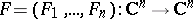be a polynomial mapping, i.e. eachis a polynomial invariables. Ifhas a polynomial mapping as an inverse, then the chain rule implies that the determinant of the Jacobi matrix is a non-zero constant. In 1939, O.H. Keller asked: is the converse true?, i.e. does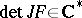imply thathas a polynomial inverse?, [a4]. This problem is now known as Keller's problem but is more often called the Jacobian conjecture. This conjecture is still open (1999) for all. Polynomial mappings satisfyingare called Keller mappings. Various special cases have been proved:

1) if, the conjecture holds (S.S. Wang). Furthermore, it suffices to prove the conjecture for alland all Keller mappings of the form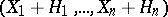where each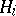is either zero or homogeneous of degree(H. Bass, E. Connell, D. Wright, A. Yagzhev). This case is referred to as the cubic homogeneous case. In fact, it even suffices to prove the conjecture for so-called cubic-linear mappings, i.e. cubic homogeneous mappings such that eachis of the form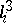, where each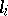is a linear form (L. Drużkowski). The cubic homogeneous case has been verified for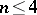(was settled by D. Wright;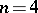was settled by E. Hubbers).

2) A necessary condition for the Jacobian conjecture to hold for allis that for Keller mappings of the form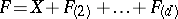with all non-zero coefficients in each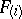positive, the mapping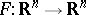is injective (cf. also Injection), wheredenotes the homogeneous part of degreeof. It is known that this condition is also sufficient! (J. Yu). On the other hand, the Jacobian conjecture holds for alland all Keller mappings of the form, where each non-zero coefficient of allis negative (also J. Yu).

3) The Jacobian conjecture has been verified under various additional assumptions. Namely, ifhas a rational inverse (O.H. Keller) and, more generally, if the field extension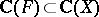is a Galois extension (L.A. Campbell). Also, properness ofor, equivalently, if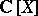is finite over(cf. also Extension of a field) implies that a Keller mapping is invertible.

4) If, the Jacobian conjecture has been verified for all Keller mappingswith(T.T. Moh) and if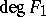or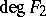is a product of at most two prime numbers (H. Applegate, H. Onishi). Finally, if there exists one linesuch that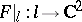is injective, then a Keller mappingis invertible (J. Gwozdziewicz). There are various seemingly unrelated formulations of the Jacobian conjecture. For example,

a) up to a polynomial coordinate change,is the only commutative-basis of;

b) every order-preserving-endomorphism of theth Weyl algebrais an isomorphism (A. van den Essen).

c) for every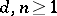there exists a constantsuch that for every commutative-algebraand everywith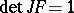and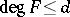, one has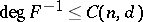(H. Bass).

d) if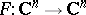is a polynomial mapping such thatfor some, thenfor some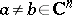.

e) if, in the last formulation, one replacesbythe so-called real Jacobian conjecture is obtained, i.e. ifis a polynomial mapping such that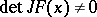for all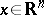, thenis injective. It was shown in 1994 (S. Pinchuk) that this conjecture is false for. Another conjecture, formulated by L. Markus and H. Yamabe in 1960 is the global asymptotic stability Jacobian conjecture, also called the Markus–Yamabe conjecture. It asserts that ifis a-mapping with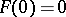and such that for allthe real parts of all eigenvalues of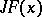are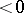, then each solution of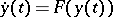tends to zero iftends to infinity. The Markus–Yamabe conjecture (for all) implies the Jacobian conjecture. Forthe Markus–Yamabe conjecture was proved to be true (R. Fessler, C. Gutierrez). However, in 1995 polynomial counterexamples where found for all(A. Cima, A. van den Essen, A. Gasull, E. Hubbers, F. Mañosas).

How to Cite This Entry:
Jacobian conjecture. Encyclopedia of Mathematics. URL: http://encyclopediaofmath.org/index.php?title=Jacobian_conjecture&oldid=19054
This article was adapted from an original article by A. van den Essen (originator), which appeared in Encyclopedia of Mathematics - ISBN 1402006098. See original article